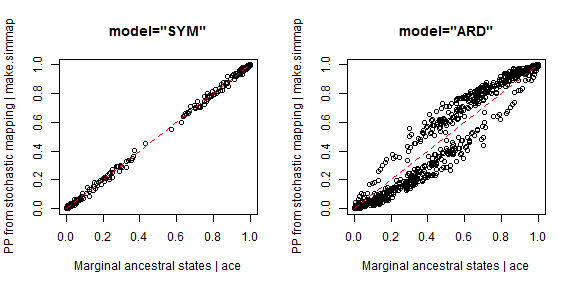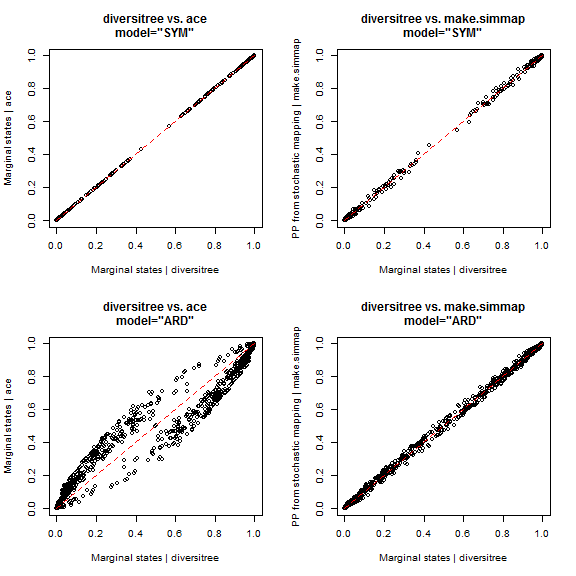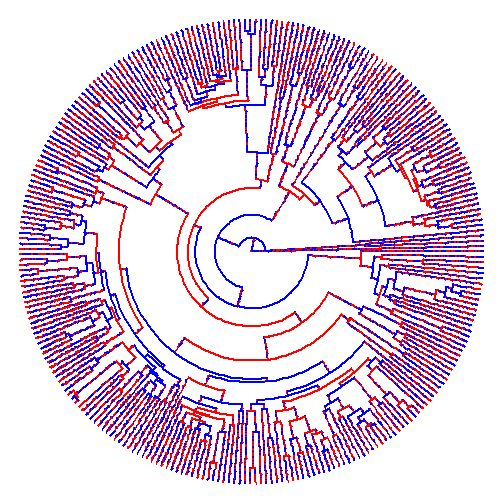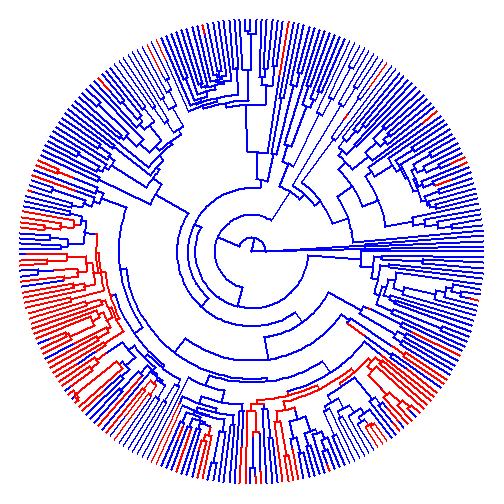## Wednesday, September 21, 2016

### Possible bug in `ace(...,model="ARD")` (and other non-symmetric models)

There appears to be a bug with `ace(...,model="ARD")`. Reversible models work fine with `ace`, ape's function for ancestral state estimation; however with asymmetric models - in which qi,j and qj,i are not necessarily equal for all i & j - something seems to be awry.

I will report this to Emmanuel, of course - but I thought that I would post the demo so that he (& others) can see what I mean.

I'm going to use an empirical dataset that was shared with me to help identify the problem; however I have removed all identifying information from the phylogeny & trait data. Note that the issue is not always nearly as readily identifiable as it is in this case. Note also that it does not have to do with model fitting, as the same non-reversible model is fit (more or less - to a reasonable standard of numerical precision) by `ace`, `make.simmap`, and diversitree. Rather the issue seems to be in the calculation of the marginal ancestral states given a particular fitted model.

``````library(phytools)
library(diversitree)

## SYM model
trees.SYM<-make.simmap(phy,x,model="SYM",nsim=500) ## make simmap
``````
``````## make.simmap is sampling character histories conditioned on the transition matrix
##
## Q =
##              0            1
## 0 -0.005818548  0.005818548
## 1  0.005818548 -0.005818548
## (estimated using likelihood);
## and (mean) root node prior probabilities
## pi =
##   0   1
## 0.5 0.5
``````
``````## Done.
``````
``````smp.SYM<-summary(trees.SYM) ## PP from stochastic mapping
fit.SYM<-ace(x,phy,type="discrete",model="SYM") ## marginal ASR

## ARD model
trees.ARD<-make.simmap(phy,x,model="ARD",nsim=500) ## make.simmap
``````
``````## make.simmap is sampling character histories conditioned on the transition matrix
##
## Q =
##              0            1
## 0 -0.026275656  0.026275656
## 1  0.008346216 -0.008346216
## (estimated using likelihood);
## and (mean) root node prior probabilities
## pi =
##   0   1
## 0.5 0.5
``````
``````## Done.
``````
``````smp.ARD<-summary(trees.ARD) ## PP from stochastic mapping
fit.ARD<-ace(x,phy,type="discrete",model="ARD") ## marginal ASR

## compare each one
par(mfrow=c(1,2))
plot(fit.SYM\$lik.anc,smp.SYM\$ace,main="model=\"SYM\"",
xlab="Marginal ancestral states | ace",
ylab="PP from stochastic mapping | make.simmap")
lines(c(0,1),c(0,1),lty="dashed",col="red")
plot(fit.ARD\$lik.anc,smp.ARD\$ace,main="model=\"ARD\"",
xlab="Marginal ancestral states | ace",
ylab="PP from stochastic mapping | make.simmap")
lines(c(0,1),c(0,1),lty="dashed",col="red")
``````These should both be the same - on average, that is. Stochastic mapping is, as the name implies, a stochastic procedure, so we might expect small differences. On average, and over a large number of replicates (as in this case; however) they should match.

It's hard to say what's wrong, here, so I thought I'd compare to diversitree which can also fit the Mk model & compute ancestral states:

``````## now compare both to diversitree
lik<-make.mk2(phy,x) ## make likelihood function
## first "SYM"
lik.sym<-constrain(lik,q01~q10)
fitted.sym<-find.mle(lik.sym,x.init=0.1)
asr.sym<-asr.marginal(lik.sym,pars=fitted.sym\$par)
## next "ARD"
fitted.ard<-find.mle(lik,x.init=c(0.1,0.1)) ## also tried root=ROOT.FLAT
asr.ard<-asr.marginal(lik,pars=fitted.ard\$par)

## compare back to ace & make.simmap
par(mfrow=c(2,2))
plot(t(asr.sym),fit.SYM\$lik.anc,
main="diversitree vs. ace\nmodel=\"SYM\"",
xlab="Marginal states | diversitree",
ylab="Marginal states | ace")
lines(c(0,1),c(0,1),lty="dashed",col="red")
plot(t(asr.sym),smp.SYM\$ace,
main="diversitree vs. make.simmap\nmodel=\"SYM\"",
xlab="Marginal states | diversitree",
ylab="PP from stochastic mapping | make.simmap")
lines(c(0,1),c(0,1),lty="dashed",col="red")
plot(t(asr.ard),fit.ARD\$lik.anc,
main="diversitree vs. ace\nmodel=\"ARD\"",
xlab="Marginal states | diversitree",
ylab="Marginal states | ace")
lines(c(0,1),c(0,1),lty="dashed",col="red")
plot(t(asr.ard),smp.ARD\$ace,
main="diversitree vs. make.simmap\nmodel=\"ARD\"",
xlab="Marginal states | diversitree",
ylab="PP from stochastic mapping | make.simmap")
lines(c(0,1),c(0,1),lty="dashed",col="red")
``````So it seems that `make.simmap` and diversitree agree for `model="ARD"` (remember, `make.simmap` is a stochastic method, so we don't expect them to match exactly, except as our number of simulations going to infinity), but that `ace` does not. That's all I can say at the moment about this, but it is probably worth looking into!

For full disclosure, this issue (identified as a possible error with `make.simmap`) was discovered by someone else & pointed out to me. (Also, initially I didn't believe it!)

## Tuesday, September 20, 2016

### Fix to pass optional `...` arguments to `fitMk` in `make.simmap`

I just pushed a tiny change to `make.simmap` which allows the optional argument `...` to be passed internally to `fitMk` for `Q="empirical"` (i.e., empirical Bayesian, in other words setting Q to its MLE).

The reason for this is that in some circumstances `fitMk`, even though it computes the likelihood no problem, will not converge on the MLE of Q which in turn can cause `make.simmap` to produce a strange result.

Case in point? The example a showed previously in which a failure to find the correct solution for Q causes the number of estimated changes using `make.simmap` to be very large (when it should in fact be quite small).

For instance:

``````library(phytools)
mtree<-make.simmap(tree,x)
``````
``````## make.simmap is sampling character histories conditioned on the transition matrix
##
## Q =
##           0         1
## 0 -1.000294  1.000294
## 1  1.000294 -1.000294
## (estimated using likelihood);
## and (mean) root node prior probabilities
## pi =
##   0   1
## 0.5 0.5
``````
``````## Done.
``````
``````plot(mtree,colors=setNames(c("blue","red"),c(0,1)),
ftype="off",type="fan")
````````````summary(mtree)
``````
``````## 1 tree with a mapped discrete character with states:
##  0, 1
##
## tree has 13731 changes between states
##
## changes are of the following types:
##      0    1
## 0    0 6836
## 1 6895    0
##
## mean total time spent in each state is:
##                 0            1    total
## raw  6873.3187518 6733.8689343 13607.19
## prop    0.5051241    0.4948759     1.00
``````
``````mtree\$logL
``````
``````##  -187.8429
``````

Whereas, when we change the optimizer for Q

``````mtree<-make.simmap(tree,x,opt.method="optim")
``````
``````## make.simmap is sampling character histories conditioned on the transition matrix
##
## Q =
##             0           1
## 0 -0.00510456  0.00510456
## 1  0.00510456 -0.00510456
## (estimated using likelihood);
## and (mean) root node prior probabilities
## pi =
##   0   1
## 0.5 0.5
``````
``````## Done.
``````
``````plot(mtree,colors=setNames(c("blue","red"),c(0,1)),
ftype="off",type="fan")
````````````summary(mtree)
``````
``````## 1 tree with a mapped discrete character with states:
##  0, 1
##
## tree has 71 changes between states
##
## changes are of the following types:
##    0  1
## 0  0 44
## 1 27  0
##
## mean total time spent in each state is:
##                 0            1    total
## raw  1.078702e+04 2820.1642667 13607.19
## prop 7.927445e-01    0.2072555     1.00
``````
``````mtree\$logL
``````
``````##  -155.9019
``````

So far I haven't encountered any problems with this - such as can occur when nested functions use optional arguments differently that happen to have the same name. Please don't hesitate to report any problems.

Good night!